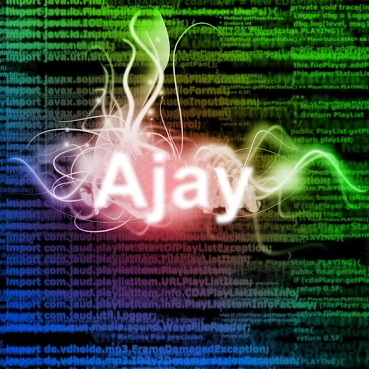Ajay Ramachandran

# Repository

https://github.com/ajayyy/VosterCoasterVR

# Automatic Curve CreationI made it so that you can automatically create curves with just moving your controller around the 3D environment. This uses the angle and position relative to the last placed track to form either a curve or a straight line.

This took on many different variations to eventually get working, so I am going to show how it currently works, as well as a summary of a few of the other ways I tried getting it working (as this post would be way too long if I showed them all.

# Other changes

I also reformatted some classes. Instead of having a specific class called AddIncline for managing track adjustments, there is now the TrackPiece class for managing all items related to each track piece, and each track piece has its own instance of the class. The RollerCoaster class then uses these classes to manage the individual track pieces.

# How you build a roller coaster in this game

To build a roller coaster, you point your controller in the direction you want the next segment of the roller coaster to point, and move the controller away from the last placed track. A track new track segment will be made between the last track segment and your controller position, ending off with the angle you are pointing at, turning if necessary.# How this is implemented

In the final iteration, I tried to make the system as simple as possible to avoid issues.

It first creates two linear equations. One for the target and one for the start. Then, finds the point of intersection

``````//calculate the slope for the target angle
float targetSlope = Mathf.Tan((90 - targetAngle.y) * Mathf.Deg2Rad);
//calculate slope for the start
float startSlope = Mathf.Tan((90 - getCurrentAngle(startTrack).y) * Mathf.Deg2Rad);

//the b value for the target angle (b = y - mx)
float targetB = rightController.transform.position.z - targetSlope * rightController.transform.position.x;
//the b value for the start angle (b = y - mx)
float startB = startTrack.transform.position.z - startSlope * startTrack.transform.position.x;

//calculate the collision point
float collisionX = (startB - targetB) / (targetSlope - startSlope);
float collisionY = targetSlope * collisionX + targetB;

//get distance from the start
float distanceFromStart = Mathf.Sqrt(Mathf.Pow(collisionX - startTrack.transform.position.x, 2)
+ Mathf.Pow(collisionY - startTrack.transform.position.z, 2));

//get distance from target
float distanceFromTarget = Mathf.Sqrt(Mathf.Pow(collisionX - rightController.transform.position.x, 2)
+ Mathf.Pow(collisionY - rightController.transform.position.z, 2));``````A curve is then added in the middle to change the angles.Lines of track straight to that collision point from the target and the start are then created, however when getting close to the middle, it creates a curve to smooth out the transition.

``````//the amount of tracks need coming straight off the start track
int startTracksNeeded = (int) Mathf.Abs(distanceFromStart / trackBoneSize / 9f);
int targetTracksNeeded = (int) Mathf.Abs(distanceFromTarget / trackBoneSize / 9f);
int curveTracksNeeded = Mathf.Min(startTracksNeeded, targetTracksNeeded);

startTracksNeeded -= curveTracksNeeded;
targetTracksNeeded -= curveTracksNeeded;

int totalTracksNeeded = startTracksNeeded + curveTracksNeeded + targetTracksNeeded;

int startTrackIndex = trackPieces.IndexOf(startTrack);
for (int i = 1; i < totalTracksNeeded + 1; i++) {

Vector3 eulerAngles = getCurrentAngle(startTrack);
//the total angle going through one whole track piece
Vector3 totalTrackAngle = Vector3.zero;

if(i > startTracksNeeded) {
//then it is time to create a curve instead of just a straight line coming off the start track
//calculate the adjustment needed for the curve
eulerAngles = angle / curveTracksNeeded * (i - 1 - startTracksNeeded) + getCurrentAngle(startTrack);

totalTrackAngle = angle / curveTracksNeeded;
}

if (i > startTracksNeeded + curveTracksNeeded) {
//back to straight path, but in the angle of the target

eulerAngles = targetAngle;

totalTrackAngle = Vector3.zero;
}

if (startTrackIndex + i < trackPieces.Count) {
GameObject trackPiece = trackPieces[i + startTrackIndex];

//reset position and angle before adjusting the track
trackPiece.transform.position = Vector3.zero;
trackPiece.transform.localEulerAngles = Vector3.zero;

//this finds the last bone plus half of the track size (because position is based off the center of the object
Vector3 modifiedPosition = trackPieces[i + startTrackIndex - 1].transform.Find("Bottom_Rail/Joint_3_3/Joint_1_3/Joint_2_4/Joint_3_4/Joint_4_3/Joint_5_3/Joint_6_3/Joint_7_3/Joint_8_3/Joint_9_3/Joint_10_3").position;

//need to offset it by trackBoneSize by the angle (for now just with y part of angle
trackPiece.transform.position = modifiedPosition - (new Vector3(Mathf.Sin(eulerAngles.y * Mathf.Deg2Rad), 0, Mathf.Cos(eulerAngles.y * Mathf.Deg2Rad)) * (trackBoneSize * 5));

//set track rotation (after adjustment to make sure the adjustment process goes well)
trackPiece.transform.localEulerAngles = eulerAngles;

} else {

//this finds the last bone plus half of the track size (because position is based off the center of the object
Vector3 modifiedPosition = trackPieces[i + startTrackIndex - 1].transform.Find("Bottom_Rail/Joint_3_3/Joint_1_3/Joint_2_4/Joint_3_4/Joint_4_3/Joint_5_3/Joint_6_3/Joint_7_3/Joint_8_3/Joint_9_3/Joint_10_3").position;

GameObject trackPiece = AddTrackPiece(totalTrackAngle, modifiedPosition, eulerAngles);

}
}``````

This creates a straight line until there have been more than the start tracks spawned, then creates the curve, then finishes with straight tracks in the target angle toward the target position.

# Reaching this point

I started out by drawing out all of the lines I was dealing with to attempt to make a curve that fits the required parameters.This helped me visualize all of the lines needed to be created to connect these two angles and where a curve could be created to create a seamless curve.

I then tried rearranging equations to get the radius of a curve by finding two points on the curve.(calculations can be found here)

I tried creating another equation to calculate the radius required to create a curve that touches two collision points, but it did not actually work in the most situations, because in most situations, one continuous curve from the start to the target is not possible, and instead there need to be a few straight tracks, then a curve, then a few more straight tracks.(Approximation of what this would look like)

However this did not actually work practically. I ended up figuring out a much simpler solution (as outlined above).

# Pull Request

https://github.com/ajayyy/VosterCoasterVR/pull/9

You can see the full set of changes in the pull request.

# GitHub Account

https://github.com/ajayyy